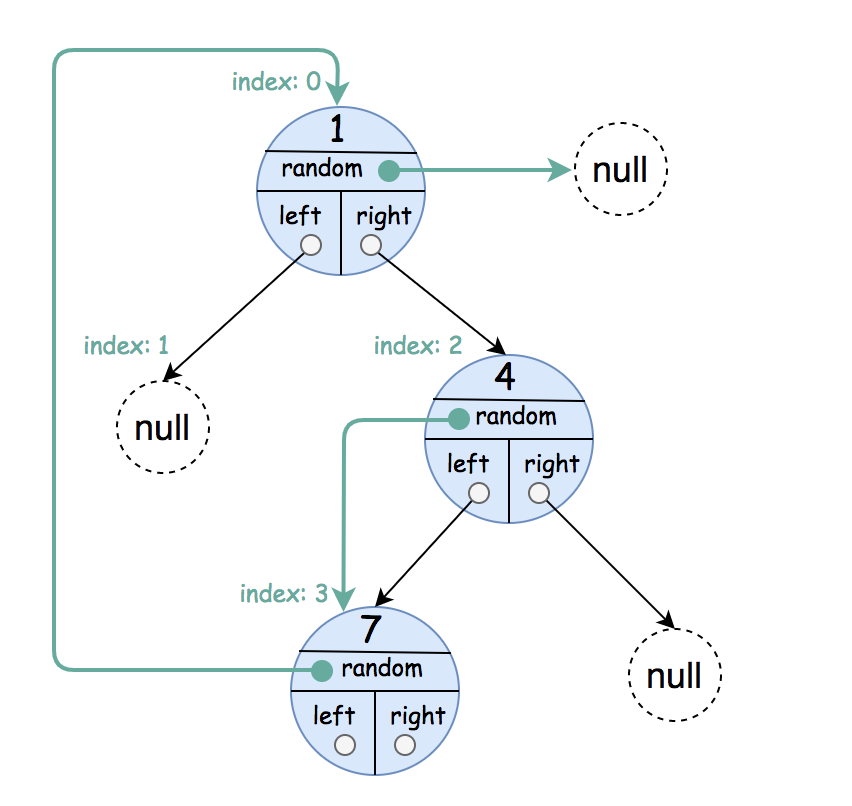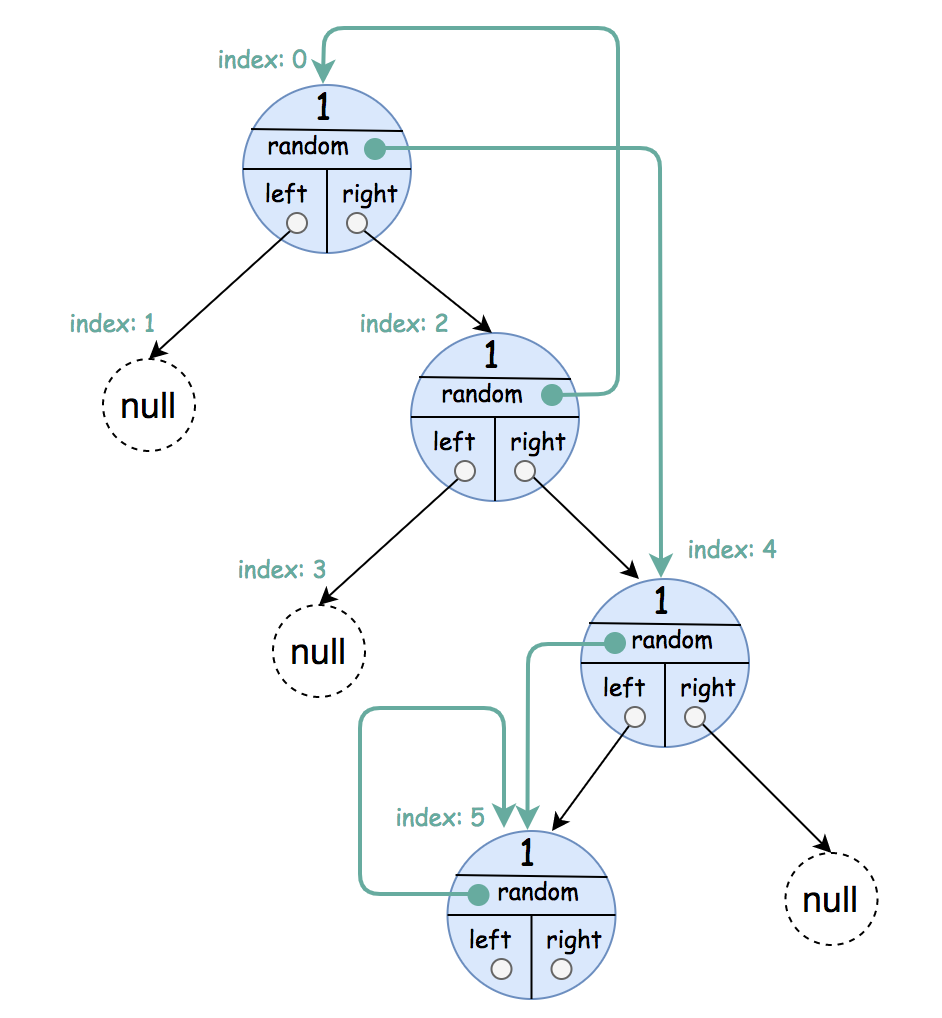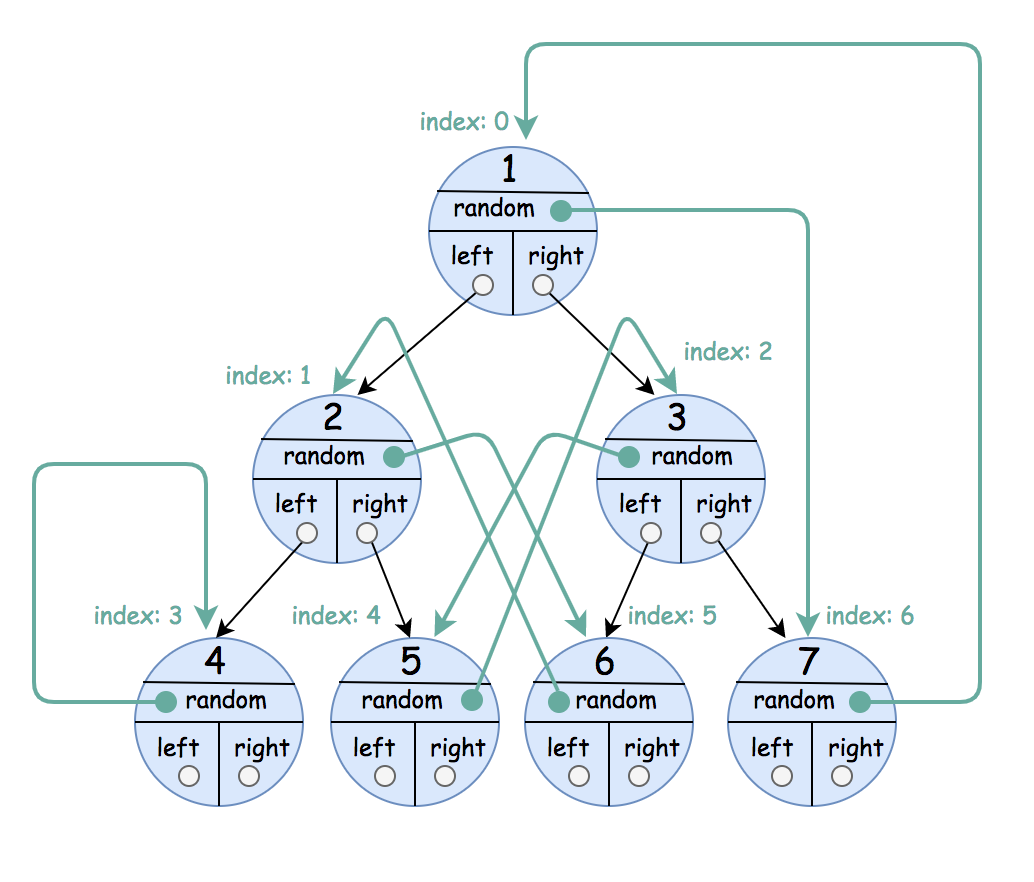# 1485. Clone Binary Tree With Random Pointer

A binary tree is given such that each node contains an additional random pointer which could point to any node in the tree or null.

Return a deep copy of the tree.

The tree is represented in the same input/output way as normal binary trees where each node is represented as a pair of `[val, random_index]` where:

• `val`: an integer representing `Node.val`
• `random_index`: the index of the node (in the input) where the random pointer points to, or `null` if it does not point to any node.

You will be given the tree in class `Node` and you should return the cloned tree in class `NodeCopy`. `NodeCopy` class is just a clone of `Node` class with the same attributes and constructors.

Example 1:```Input: root = [[1,null],null,[4,3],[7,0]]
Output: [[1,null],null,[4,3],[7,0]]
Explanation: The original binary tree is [1,null,4,7].
The random pointer of node one is null, so it is represented as [1, null].
The random pointer of node 4 is node 7, so it is represented as [4, 3] where 3 is the index of node 7 in the array representing the tree.
The random pointer of node 7 is node 1, so it is represented as [7, 0] where 0 is the index of node 1 in the array representing the tree.
```

Example 2:```Input: root = [[1,4],null,[1,0],null,[1,5],[1,5]]
Output: [[1,4],null,[1,0],null,[1,5],[1,5]]
Explanation: The random pointer of a node can be the node itself.
```

Example 3:```Input: root = [[1,6],[2,5],[3,4],[4,3],[5,2],[6,1],[7,0]]
Output: [[1,6],[2,5],[3,4],[4,3],[5,2],[6,1],[7,0]]
```

Example 4:

```Input: root = []
Output: []
```

Example 5:

```Input: root = [[1,null],null,[2,null],null,[1,null]]
Output: [[1,null],null,[2,null],null,[1,null]]
```

Constraints:

• The number of nodes in the `tree` is in the range `[0, 1000].`
• Each node's value is between `[1, 10^6]`.

Medium

Prime

Amazon

### Solution(Chinese):

LEETCODE 1485. Clone Binary Tree With Random Pointer 解题思路分析